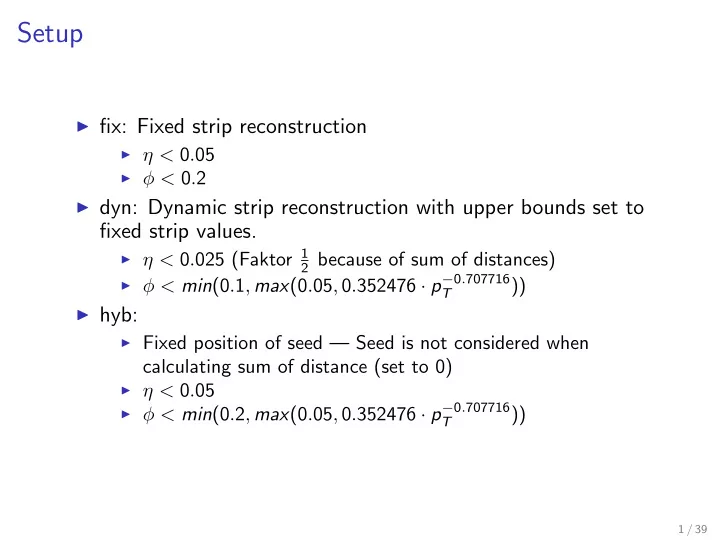# Setup fix: Fixed strip reconstruction < 0 . 05 < 0 . 2 - PowerPoint PPT Presentation

## Setup fix: Fixed strip reconstruction < 0 . 05 < 0 . 2 dyn: Dynamic strip reconstruction with upper bounds set to fixed strip values. < 0 . 025 (Faktor 1 2 because of sum of distances) < min (0 . 1 ,

1. Setup ◮ fix: Fixed strip reconstruction ◮ η < 0 . 05 ◮ φ < 0 . 2 ◮ dyn: Dynamic strip reconstruction with upper bounds set to fixed strip values. ◮ η < 0 . 025 (Faktor 1 2 because of sum of distances) ◮ φ < min (0 . 1 , max (0 . 05 , 0 . 352476 · p − 0 . 707716 )) T ◮ hyb: ◮ Fixed position of seed — Seed is not considered when calculating sum of distance (set to 0) ◮ η < 0 . 05 ◮ φ < min (0 . 2 , max (0 . 05 , 0 . 352476 · p − 0 . 707716 )) T 1 / 39

2. ZTT fixed strp 2 / 39

3. ZTT dynamic strip 3 / 39

4. ZTT hybrid strip 4 / 39

5. ZTT fixed strp 5 / 39

6. ZTT dynamic strip 6 / 39

7. ZTT hybrid strip 7 / 39

8. ZTT fixed strp 8 / 39

9. ZTT dynamic strip 9 / 39

10. ZTT hybrid strip 10 / 39

11. QCD fixed strp 11 / 39

12. QCD dynamic strip 12 / 39

13. QCD hybrid strip 13 / 39

14. QCD fixed strp 14 / 39

15. QCD dynamic strip 15 / 39

16. QCD hybrid strip 16 / 39

17. QCD fixed strp 17 / 39

18. QCD dynamic strip 18 / 39

19. QCD hybrid strip 19 / 39

20. ROC curves for 20 < p T ( τ ) < 100: p T ( outer ) / p reco T 20 / 39

21. ROC curves for 20 < p T ( τ ) < 30: p T ( outer ) / p reco T 21 / 39

22. ROC curves for 30 < p T ( τ ) < 40: p T ( outer ) / p reco T 22 / 39

23. ROC curves for 40 < p T ( τ ) < 100: p T ( outer ) / p reco T 23 / 39

24. ROC curves for 20 < p T ( τ ) < 100: neutral Iso 24 / 39

25. ROC curves for 20 < p T ( τ ) < 30: neutral Iso 25 / 39

26. ROC curves for 30 < p T ( τ ) < 40: neutral Iso 26 / 39

27. ROC curves for 40 < p T ( τ ) < 100: neutral Iso 27 / 39

28. ROC curves for 20 < p T ( τ ) < 100: charged Iso 28 / 39

29. ROC curves for 20 < p T ( τ ) < 30: charged Iso 29 / 39

30. ROC curves for 30 < p T ( τ ) < 40: charged Iso 30 / 39

31. ROC curves for 40 < p T ( τ ) < 100: charged Iso 31 / 39

32. ROC curves for 20 < p T ( τ ) < 100: comb Iso 32 / 39

33. ROC curves for 20 < p T ( τ ) < 30: comb Iso 33 / 39

34. ROC curves for 30 < p T ( τ ) < 40: comb Iso 34 / 39

35. ROC curves for 40 < p T ( τ ) < 100: comb Iso 35 / 39

36. ROC curves for 20 < p T ( τ ) < 100: comb Iso + p T ( outer ) cut 36 / 39

37. ROC curves for 20 < p T ( τ ) < 30: comb Iso + p T ( outer ) cut 37 / 39

38. ROC curves for 30 < p T ( τ ) < 40: comb Iso + p T ( outer ) cut 38 / 39

39. ROC curves for 40 < p T ( τ ) < 100: comb Iso + p T ( outer ) cut 39 / 39

More recommend